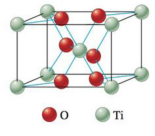Chapter 21, Problem 28E

Chapter
Section
Textbook Problem

# Titanium dioxide, the most widely used white pigment, occurs naturally but is often colored by the presence of impurities. The chloride process is often used in purifying rutile, a mineral form of titanium dioxide.a. Show that the unit cell for rutile, shown below, conforms to the formula TiO2. (Hint: Recall the discussion in Sections 9-4 and 9-7.)b. The reactions for the chloride process are 2TiO 2 ( s )   +  3C ( s )   +  4Cl 2 ( g ) → 950 ∘ C 2TiCl 4 ( g )   +  CO 2 ( g )   +  2CO ( g ) TiCl 4 ( g )   +  O 2 ( g ) → 1000 − 1400 ∘ C TiO 2 ( s )   +  2Cl 2 ( g ) Assign oxidation states to the elements in both reactions. Which elements are being reduced, and which are being oxidized? Identify the oxidizing agent and the reducing agent in each reaction.

(a)

Interpretation Introduction

Interpretation: It is to be shown that the given structure conforms to the formula of TiO2 . The oxidation states of each element in the given reactions are to be assigned. The elements that are reduced and the elements that are oxidized in the given reactions are to be identified. The oxidizing agent and reducing agent in these reactions are to be identified.

Concept introduction: The substance that gains electron (oxidation number increases) is known as oxidizing agent, whereas the substance that loses electron (oxidation number decreases) is known as reducing agent.

Loss of Oxygen atom is known as reduction, whereas gain of Oxygen is known as oxidation.

Explanation

EXPLANATION

The given structure conforms to the formula of TiO2 .

The given structure is,

Figure 1

In the above figure,

• Red color represents Oxygen (O) atom.
• Green color represents Titanium (Ti) atom.

In the above diagram, Titanium atoms are arranged in BCC array, where eight Ti atoms are arranged at eight corners and one Ti atom is at body center. The number of Ti atoms is calculated using the formula.

NumberofTiatoms=((8corners×18percorneratom)+Total body centered atom)

Substitute the value of total body centered Ti atoms in the above equation

(b)

Interpretation Introduction

Interpretation: It is to be shown that the given structure conforms to the formula of TiO2 . The oxidation states of each element in the given reactions are to be assigned. The elements that are reduced and the elements that are oxidized in the given reactions are to be identified. The oxidizing agent and reducing agent in these reactions are to be identified.

Concept introduction: The substance that gains electron (oxidation number increases) is known as oxidizing agent, whereas the substance that loses electron (oxidation number decreases) is known as reducing agent.

Loss of Oxygen atom is known as reduction, whereas gain of Oxygen is known as oxidation.

To determine: The oxidation states of each element in the given reactions; the elements that are reduced and the elements that are oxidized in the given reaction.; the oxidizing agent and reducing agent in these reactions.

### Still sussing out bartleby?

Check out a sample textbook solution.

See a sample solution

#### The Solution to Your Study Problems

Bartleby provides explanations to thousands of textbook problems written by our experts, many with advanced degrees!

Get Started